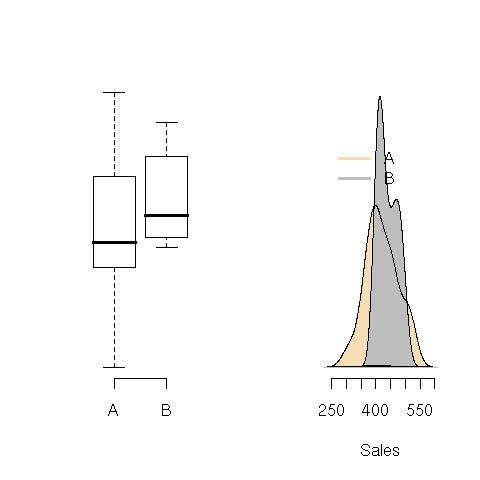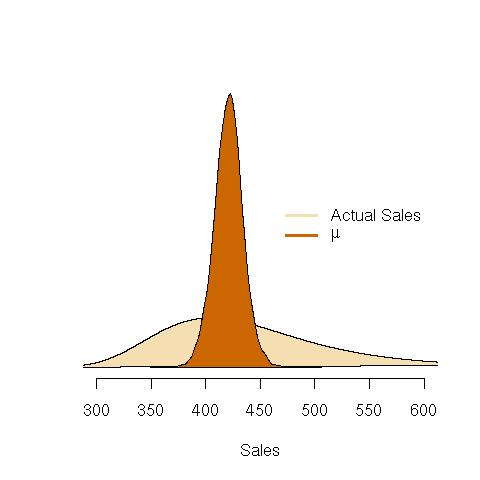# Why most statistics don’t mean what you think they do: Part I.

Here’s a common, classical statistics problem. Uncle Ted’s chain of Kill ’em and Grill ’em Venison Burgers tested two ad campaigns, A and B, and measured the sales of sausage sandwiches for 20 days under both campaigns. This was done, and it was found that mean(A) = 421, and mean(B) = 440. The question is: are the campaigns different?

In Part II of this post, I will ask the following, which is not a trick question: what is the probability that mean(A) < mean(B)? The answer will surprise you. But for right now, I merely want to characterize the sales of sausages under Campaigns A and B. Rule #1 is always look at your data! So we start with some simple plots:I will explain box and density plots elsewhere; but for short: these pictures show the range and variability of the actual observed sales for the 20 days of the ad campaigns. Both plots show the range and frequency of the sales, but show it in different ways. Even if you don’t understand these plots well, you can see that the sales under the two campaigns was different. Let’s concentrate on Campaign A.

This is where it starts to get hard, because we first need to understand that, in statistics, data is described by probability distributions, which are mathematical formulas that characterize pictures like those above. The most common probability distribution is the normal, the familiar bell-shaped curve.

The classical way to begin is to then assume that the sales, in A (and B too), follow a normal distribution. The plots give us some evidence that this assumption is not terrible—the data is sort of bell-shaped—but not perfectly so. But this slight deviation from the assumptions is not the problem, yet.

A normal distribution needs two numbers, or parameters, to fully describe it: call them ? and ?^2. We use Greek letters to denote that we do not know the actual values of these parameters. We have to guess them. To indicate the guess, we usually put a “hat” on top of the letter. Here, we can call the guess ? (we also have to guess ?, but we’ll ignore that problem here).

The value of ? = mean(A) = 421. Now, we do not know that ? exactly equals 421, that is just our best guess. Since it is a guess, it could be wrong. ? might actually equal 422 or 420, or some other number. So we have to find some way to express our uncertainty in this guess.

The classical way to do this is through a confidence interval, probably the most bizarre creation of old-time statistics (which is a story for another time). The modern (or Bayesian) interpretation is called a credible interval. It is a range of numbers, here [395, 447], such that we can say there is a 95% chance that the actual value of ? is in that interval (given the evidence we have from the 20 days of data).

OK so far? Because here it gets even harder. It turns out that we can draw a picture of the probability that ? takes any particular value. This is the same kind of picture as the one we drew above for the observed sales data in the two Campaigns (the picture on the right). I’ll draw that in a second, but first the main point of this article:

The confidence or credible interval does not mean that there is a 95% chance that the sales in Campaign A will be between 395 to 447!

It does mean that there is a 95% chance that the parameter ? will be between 395 to 447. But the actual sales of A will be entirely different. This is a crucial distinction to make, and one that is often forgotten. The following picture, which shows both the probability distribution of ? and future sales, demonstrates what I mean:Notice that the probability distribution of ? is much narrower than that of future sales. This means that we are far, far less certain about what actual future sales of A will be than we are about the value of ?. For example, the probability that ? > 500 is nearly 0, but the chance that future sales of A are larger than 500 is nearly 20%!

But people make this kind of mistake all the time. They speak of the parameters of probability models like they are the actual observable entities. So, this long-winded introduction finally leads us to our lesson: parameters of probability models, while necessary to create models in the first place, are unobservable, and do not directly correspond to reality. They are nearly always meaningless in and of themselves, and they must be tied back to some observable quantity (like sales).

The main problem is that nearly all statistical methods are centered around making statements about unobservable parameters, when in reality, people want to know about observable numbers like sales. There is a branch of statistics, called “Predictive Statistics”, that is starting to turn this around, but it’s a relatively new and under-explored.

In future posts, I’ll give examples of this common mistake. By far the most frequent is the so-called “difference between means test” that we started this lesson with. Namely, did the sales data from the two Campaigns actually differ? Stay tuned!

Posted in Uncategorized

## 2 Thoughts

1.TCO says:

Is this the mistake that Beaker is calling out wrt Douglas paper on the CA thread?

R u Beaker?

2.TCO says:

There’s a bunch of things that I don’t understand about your example. I know you just set it up quick to make a different point, but it still bugs me that I don’t understand.

Are the numbers that are being displayed, sales per day or total sales per store or per trial of the campaign or what? If it’s sales per day, does it really make sense to treat them as independant (as there will be meaningful trends with consumer awareness and such).

How were the different trials seperated? Were they run in different regions? Or one after the other in the same one? Each of these gives a confounding variable. If we imagine different stores, is there still some chance that the tests may interact with each other (through consumer awareness)?

Why are there such nice smooth curves rather than histograms?